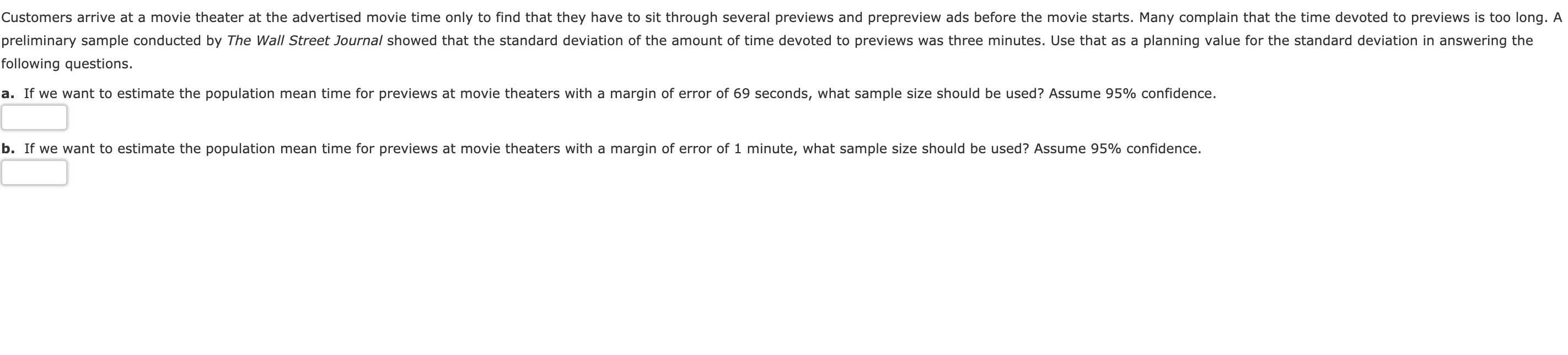# Customers arrive at a movie theater at the advertised movie time only to find that they have to sit through several previews and prepreview ads before the movie starts. Many complain that the time devoted to previews is too long. Apreliminary sample conducted by The Wall Street Journal showed that the standard deviation of the amount of time devoted to previews was three minutes. Use that as a planning value for the standard deviation in answering thefollowing questions.a. If we want to estimate the population mean time for previews at movie theaters with a margin of error of 69 seconds, what sample size should be used? Assume 95% confidence.b. If we want to estimate the population mean time for previews at movie theaters with a margin of error of 1 minute, what sample size should be used? Assume 95% confidence.

Question
5473 viewshelp_outlineImage TranscriptioncloseCustomers arrive at a movie theater at the advertised movie time only to find that they have to sit through several previews and prepreview ads before the movie starts. Many complain that the time devoted to previews is too long. A preliminary sample conducted by The Wall Street Journal showed that the standard deviation of the amount of time devoted to previews was three minutes. Use that as a planning value for the standard deviation in answering the following questions. a. If we want to estimate the population mean time for previews at movie theaters with a margin of error of 69 seconds, what sample size should be used? Assume 95% confidence. b. If we want to estimate the population mean time for previews at movie theaters with a margin of error of 1 minute, what sample size should be used? Assume 95% confidence. fullscreen
check_circle

Step 1

a.Computing the sample size:

The sample standard deviation is 3 minutes, confidence level is 95%, and the margin of error is 69 seconds (69/60=1.15 minute). The formula for margin of error and sample size is given below:

Step 2

Here α = 0.05, E = 1.15, and s =3

From the EXCEL, using the formula, “= NORM.S.INV (0. 975)”, the z-value is 1.96.

Therefore, the sample size n can be obtained as follows:

Step 3

The required sample size is 27.

b. Computing the sample size:

The sample standard deviation is 3 minute. Assume that confidence level is 95%. The margin of error is 1 minute.  That is, α = 0.05, E = 1, and s =3

Fro...

### Want to see the full answer?

See Solution

#### Want to see this answer and more?

Solutions are written by subject experts who are available 24/7. Questions are typically answered within 1 hour.*

See Solution
*Response times may vary by subject and question.
Tagged in
MathStatistics

### Other